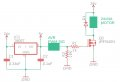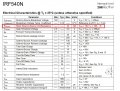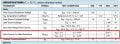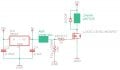# which circuit is stable PWM motor speed control using IRF540(FET)?

#### Kim-JiHoon

Joined May 3, 2020
55
Hello I have tow circuit about 'PWM DC motor speed control' and i want to know which one is more stable

my motor spec is 24v/4a (more than 2A less than 4A) for running fan.

here is circuit

CIRCUIT A)AVR PWM -> ATMEGA128 or ATMEGA series
R1 -> to gate
R2 -> for fully off
D1 -> 1N5401 (3A 100V)

CIRCUIT B)AVR PWM -> ATMEGA128 or ATMEGA series
R1 -> to TR
R2 -> for fully off
R3 -> (for protect NPN ?)
R4 -> to gate
D1 -> 1N5401 (3A 100V)
C5 -> filtering High frequency (0.1uF -> 1000uF)

I think 'B' is better than 'A' becase using two TR, 7805 and IRF540 will have less heat

but circuit 'A' is more simple.

so this is my question.

1. which one is more efficient 'A' with heatsink, 'B' without heatsink?

2. If i take 'B' is these R value is fit to this circuit?

#### Attachments

• 125.1 KB Views: 1

Joined Jul 18, 2013
21,986
Circuit A should be ample, this is the way the Typical PWM treadmill controllers do it.
Max.

#### Kim-JiHoon

Joined May 3, 2020
55
Circuit A should be ample, this is the way the Typical PWM treadmill controllers do it.
Max.

you mean Circuit A need ample (like npn, pnp TR)?

than i should take circuit B !

and resistors in circuit B is these value fit to circuit B?

#### Alec_t

Joined Sep 17, 2013
11,797
Your thread title mentions IRF540, but the circuit needs the IRFL540 version (as you correctly show) if the gate switching voltage is only 5V.

#### Kim-JiHoon

Joined May 3, 2020
55
Your thread title mentions IRF540, but the circuit needs the IRFL540 version (as you correctly show) if the gate switching voltage is only 5V.
oh i'm sorry my mistake

if i use Logic level FET (not add regulator for high voltage gate)

than IRFL540 is right? not IRF540

Joined Jul 18, 2013
21,986

you mean Circuit A need ample (like npn, pnp TR)?

than i should take circuit B !
Ample, sufficient, OK.
Circuit A.
Max.

#### Kim-JiHoon

Joined May 3, 2020
55
Your circuits have 1k gate resistance which will turn on slowly. I changed to 10 ohms.
I am concerned that the MOSFET is not turned on well. (low gate voltage)
In this circuit the Gate voltage will be about 14 volts. (not critical, could use a 12V Zener)

is it right?Then if i use IRF540N or other FET like need Rds 10v

this circuit will fit to me right?Last edited:

#### ronsimpson

Joined Oct 7, 2019
1,122
Changed bottom transistor to 2N2222A. D3 voltage in the 10 to 20V range.
Note, there is a inversion from AVR to Q1-Gate. You will need to fix this in software. At the AVR pin, 1=Q1 off. 0= Q1 on.
Most MOSFETs have a max gate voltage of +/-20V but some are 15V.
Higher voltage on the Gate will allow you to use more types of MOSFETs.
At power up, AVR out pins will be at tri-state. R2 will pull up and turn off Q1. After some instructions the AVR will take control of the I/O and you can make the pin do what you want.Joined Jul 18, 2013
21,986

Joined Jan 15, 2015
5,714
I vote for A using a suitable Logic Level MOSFET.

Ron

•#### Kim-JiHoon

Joined May 3, 2020
55

if i use Logic Level MOSFET it comes to high temp issue

so i have to find other MOSFET

and if i use other MOSFET to low temp, other MOSFET need Higher voltage on the Gate !

so this is my conclusion

1. Logic Level MOSFET is simple but limited MOSFET output voltage

2. if i need MOSFET output voltage high , that MOSFET need higher voltage on the gate

- Does not matter with MOSFET temp or low output -> Logic Level MOSFET (ex IRL series)

- Does matter with MOSFET temp or high output -> other MOSFET which need higher voltage on the Gate (ex IRF series)

•Danko

Joined Jan 15, 2015
5,714
1. Logic Level MOSFET is simple but limited MOSFET output voltage

2. if i need MOSFET output voltage high , that MOSFET need higher voltage on the gate
Generally when choosing a MOSFET it is wise to read the data sheet. A 24 volt motor is hardly high voltage. Any number of logic level MOSFETs would do just fine. If things are getting hot you have something wired incorrectly or your motor is drawing in excess of 4.0 amps. Read and understand data sheets.

Ron

#### Kim-JiHoon

Joined May 3, 2020
55
Generally when choosing a MOSFET it is wise to read the data sheet. A 24 volt motor is hardly high voltage. Any number of logic level MOSFETs would do just fine. If things are getting hot you have something wired incorrectly or your motor is drawing in excess of 4.0 amps. Read and understand data sheets.

Ron

Actually i don't fully understand data sheet.

I know difference between LOGIC-LEVEL MOSFET and the other MOSFET

and that is R(ds) on voltage.

I found some LOGIC-LEVEL MOSFET but they are like this

R(ds) on voltage 4.5v -> 2.6A

R(ds) on voltage 10v -> 3.5A

so, i can't provide 10v to LOGIC-LEVEL MOSFET with just AVR PWM

and I don't know temp, if LOGIC-LEVEL MOSFET's output is 4A (when MOSFET's MAXIMUM A is 4A)

then, if i use IRL540 like thisis this circuit work?Joined Jul 18, 2013
21,986

#### Alec_t

Joined Sep 17, 2013
11,797
I know difference between LOGIC-LEVEL MOSFET and the other MOSFET
and that is R(ds) on voltage.
R(ds) is a resistance, not a voltage.
Vgs(thr) is the usual distinguishing parameter.